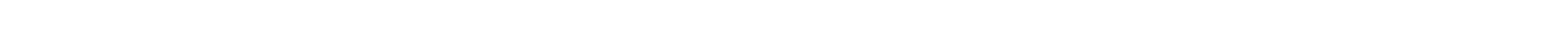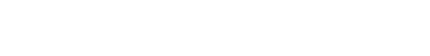# Polymorphism in C#

The name polymorphism implies one word having many forms. In programming, this means that an object can have multiple functionalities. Polymorphism is one of the fundamental concepts in object-oriented programming.

There are mainly two types of polymorphism i.e static polymorphism and dynamic polymorphism. Details about these are given as follows:

## Static Polymorphism

Static polymorphism or early binding involves linking a function with an object during compile time. The two major techniques to implement static polymorphism are function overloading and operator overloading. Details about these techniques are given as follows:

In function overloading, there can be multiple definitions for the same function name. All of these definitions may differ by the number of arguments they contain or the type of these arguments.

At the compile time, the number and type of arguments passed are checked by the compiler and on that basis a function is called. There is an error if there is no function matching the parameter list.

using System;
{
class Example
{
void printData(int rno)
{
Console.WriteLine("Roll number: {0}", rno );
}
void printData(int rno, string name)
{
Console.WriteLine("Roll number: {0}", rno );
Console.WriteLine("Name: {0}", name );
}
void printData(int rno, string name, double marks)
{
Console.WriteLine("Roll number: {0}", rno );
Console.WriteLine("Name: {0}", name );
Console.WriteLine("Marks: {0}", marks );
}
static void Main(string[] args)
{
Example obj = new Example();
obj.printData(1);
obj.printData(2, "John");
obj.printData(3, "Sally", 78.5);
}
}
}

The output of the above program is as follows:

Roll number: 1
Roll number: 2
Name: John

Roll number: 3
Name: Sally
Marks: 78.5

Now let us understand the above program.

In the above program, the function printData() is overloaded. The function that is called depends on whether there is one int argument, two int and string arguments or three int, string and double arguments. The code snippet for this is as follows:

 void printData(int rno)
{
Console.WriteLine("Roll number: {0}", rno );
}
void printData(int rno, string name)
{
Console.WriteLine("Roll number: {0}", rno );
Console.WriteLine("Name: {0}", name );
}
void printData(int rno, string name, double marks)
{
Console.WriteLine("Roll number: {0}", rno );
Console.WriteLine("Name: {0}", name );
Console.WriteLine("Marks: {0}", marks );
}

In the function Main(), first an object is created of class Example. Then the function printData() is called with various arguments. The code snippet for this is as follows:

 Example obj = new Example();
obj.printData(1);
obj.printData(2, "John");
obj.printData(3, "Sally", 78.5);

Many of the operators in C# can be overloaded. Overloaded operators are functions that contain the keyword operator before the particular operator that is being overloaded. Some of the operators that can be overloaded are unary operators (+, -, !, ++, --) , binary operators(+, -, *, /, %) etc.

using System;
{
class Point
{
private int x;
private int y;
public Point(int x1 = 0, int y1 = 0)
{
x = x1;
y = y1;
}
public static Point operator+ (Point p1, Point p2)
{
Point p3 = new Point();
p3.x = p1.x + p2.x;
p3.y = p1.y + p2.y;
return p3;
}
public void display()
{
Console.WriteLine("x = {0}", x);
Console.WriteLine("y = {0}", y);
}
}
class Example
{
static void Main(string[] args)
{
Point p1 = new Point(5, 7);
Point p2 = new Point(9, 2);
Point p3 = new Point();
p3 = p1 + p2;
Console.WriteLine("Point p1 is:");
p1.display();
Console.WriteLine("Point p2 is:");
p2.display();
Console.WriteLine("Point p3 is:");
p3.display();
}
}
}

The output of the above program is as follows:

Point p1 is:
x = 5
y = 7
Point p2 is:
x = 9
y = 2
Point p3 is:
x = 14
y = 9

Now let us understand the above program.

There are two int variables x and y in the class Point. The constructor initializes the values of x and y. The function Point operator+ implements the addition operator. It adds the x and y values for points p1 and p2 and returns the Point object p3. The function display() prints the values of x and y. The code snippet for this is given below:

class Point
{
private int x;
private int y;
public Point(int x1 = 0, int y1 = 0)
{
x = x1;
y = y1;
}
public static Point operator+ (Point p1, Point p2)
{
Point p3 = new Point();
p3.x = p1.x + p2.x;
p3.y = p1.y + p2.y;
return p3;
}
public void display()
{
Console.WriteLine("x = {0}", x);
Console.WriteLine("y = {0}", y);
}
}

The function Main() contains three objects of class Point. The objects p1 and p2 are added using operator overloading and the sum is stored in p3. Finally, the values of p1, p2 and p3 are displayed. The code snippet for this is given below:

static void Main(string[] args)
{
Point p1 = new Point(5, 7);
Point p2 = new Point(9, 2);
Point p3 = new Point();
p3 = p1 + p2;
Console.WriteLine("Point p1 is:");
p1.display();
Console.WriteLine("Point p2 is:");
p2.display();
Console.WriteLine("Point p3 is:");
p3.display();
}

## Dynamic Polymorphism

Dynamic polymorphism or late binding is implemented using abstract classes and virtual functions. Abstract classes are base classes with partial implementation. These classes contain virtual functions that are inherited by other classes that provide more functionality.

Virtual functions are implemented in a different manner for different inherited classes. The call to these virtual functions is decided at run time.

A program that demonstrates dynamic polymorphism is given as follows:

Source Code: Program that demonstrates dynamic polymorphism in C#

using System;
namespace AbstractionDemo
{
class Shape
{
public virtual double area()
{
return 0;
}
}
class Circle: Shape
{
public Circle( double r)
{
}
public override double area ()
{
}
}
class Square: Shape
{
private double side;
public Square( double s)
{
side = s;
}
public override double area ()
{
return (side*side);
}
}
class Triangle: Shape
{
private double tbase;
private double theight;
public Triangle( double b, double h)
{
tbase = b;
theight = h;
}
public override double area ()
{
return (0.5*tbase*theight);
}
}
class Test
{
static void Main(string[] args)
{
Circle c = new Circle(2.5);
Console.WriteLine("Area of Circle = {0}", c.area());
Square s = new Square(5.5);
Console.WriteLine("Area of Square = {0}", s.area());
Triangle t = new Triangle(3.0, 5.0);
Console.WriteLine("Area of Triangle = {0}", t.area());
}
}
}

The output of the above program is as follows:

Area of Circle = 19.625
Area of Square = 30.25
Area of Triangle = 7.5

Now let us understand the above program.

The class shape contains the virtual method area(). The code snippet for this is given as follows:

 class Shape
{
public virtual double area()
{
return 0;
}
}

The classes Circle, Square and Triangle inherit from Shape. Class Circle has a private data member radius. The class Square has a private data member side. Similarly, the class Triangle has private the data members tbase and theight.

All of these three classes first initialize their data members using constructors and then return the area using the function area(). The code snippet for this is given as follows:

class Circle: Shape
{
public Circle( double r)
{
}
public override double area ()
{
}
}
class Square: Shape
{
private double side;
public Square( double s)
{
side = s;
}
public override double area ()
{
return (side*side);
}
}
class Triangle: Shape
{
private double tbase;
private double theight;
public Triangle( double b, double h)
{
tbase = b;
theight = h;
}
public override double area ()
{
return (0.5*tbase*theight);
}
}

The function Main() contains the objects c, s and t for classes Circle, Square and Triangle respectively. Then the areas of the circle, square and triangle are printed using the function area(). The code snippet for this is given as follows:

 class Test
{
static void Main(string[] args)
{
Circle c = new Circle(2.5);
Console.WriteLine("Area of Circle = {0}", c.area());
Square s = new Square(5.5);
Console.WriteLine("Area of Square = {0}", s.area());
Triangle t = new Triangle(3.0, 5.0);
Console.WriteLine("Area of Triangle = {0}", t.area());
}
}#### austin faith

Avery good write-up. Please let me know what are the types of C# libraries used for AI development.#### kariya arti

very satisfied!!#### jean-Francois Michaud

Good tutorial. Small question: Say, there is : enum numbers { one, two, three} and a string field_enum ="one" how would I from the variable field_enum have a response with value numbers.one so that it can be treated as an enum and not as a string. making a list from the enum, and loop into the list. is not elegant... and may not work is forced value on field is forced ( one = 100).#### Kshitiz

Hi Team Knowledge Hut, Thank you for such an informative post like this. I am completely new to this digital marketing field and do not have much idea about this, but your post has become a supportive pillar for me. After reading the blog I would expect to read more about the topic. I wish to get connected with you always to have updates on these sorts of ideas. Regards, Kshitiz#### Ed

The reason abstraction can be used with this example is because, the triangle, circle. Square etc can be defined as a shape, for example.....shape c = new circle(5,0)...the abstract object c now points at the circle class. Thus hiding implementation

## Swift Tutorial

Introduction to Swift Tutorial

R Programming

Python Tutorial